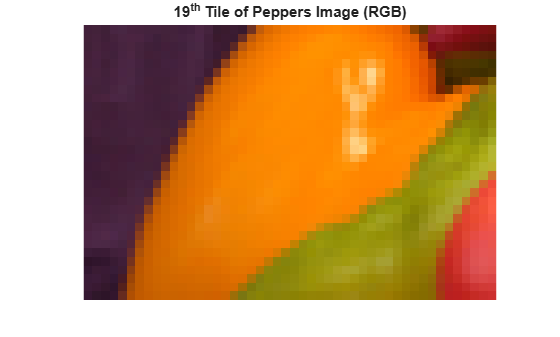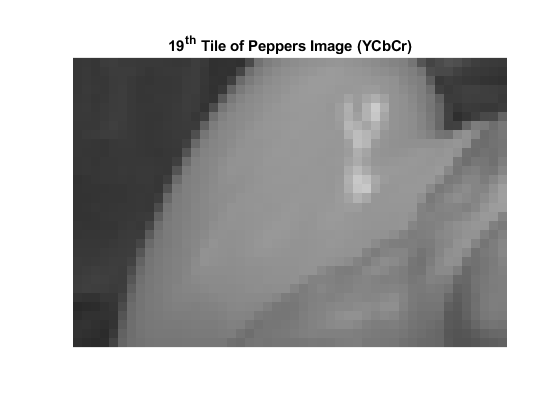## Syntax

``tileData = readEncodedTile(t,tileNumber)``
``[Y,Cb,Cr] = readEncodedTile(t,tileNumber)``

## Description

example

````tileData = readEncodedTile(t,tileNumber)` returns image data contained in the tile specified by `tileNumber` from the TIFF file represented by the `Tiff` object `t`.The `readEncodedTile` function trims the area in a tile that is outside of the `ImageLength` and `ImageWidth` boundaries. Therefore, image data from tiles that occur on the right edge or the bottom edge of the image can have different dimensions.```

example

````[Y,Cb,Cr] = readEncodedTile(t,tileNumber)` returns the YCbCr components of the image data contained in the tile specified by `tileNumber` from the TIFF file represented by the `Tiff` object `t`. Use this syntax only with images that have a YCbCr photometric interpretation.Depending upon the values of the `YCbCrSubSampling` tag, the size of the `Cb` component can differ from the `Y` component.```

## Examples

collapse all

Read a tile of image data from a TIFF file that contains an image with a tiled layout.

`t = Tiff('peppers_RGB_tiled.tif','r');`

Determine the number of tiles and the tile size in the image.

`nTiles = numberOfTiles(t)`
```nTiles = 36 ```
`tileSize = [getTag(t,'TileLength') getTag(t,'TileWidth')]`
```tileSize = 1×2 32 48 ```

Read and display the `19`th tile of the image. The `readEncodedTile` function trims the area in a tile that is outside of the `ImageLength` and `ImageWidth` boundaries. Therefore, image data from tiles that occur on the right edge or the bottom edge of the image can have different dimensions.

```tile = readEncodedTile(t,19); imshow(tile,'InitialMagnification','fit'); % Magnify for display title('19^{th} Tile of Peppers Image (RGB)');```Close the `Tiff` object.

`close(t); `

Read a tile of image data from a YCbCr TIFF file that contains an image with a tiled layout.

`t = Tiff('peppers_YCbCr_tiled.tif','r');`

Determine the number of tiles and the tile size in the image.

`nTiles = numberOfTiles(t)`
```nTiles = 36 ```
`tileSize = [getTag(t,'TileLength') getTag(t,'TileWidth')]`
```tileSize = 1×2 32 48 ```

Read and display the Y component of the `19`th tile of the image. The `readEncodedTile` function trims the area in a tile that is outside of the `ImageLength` and `ImageWidth` boundaries. Therefore, image data from tiles that occur on the right edge or the bottom edge of the image can have different dimensions.

```[Y,Cb,Cr] = readEncodedTile(t,19); imshow(Y,'InitialMagnification','fit') % Magnify for display title('19^{th} Tile of Peppers Image (YCbCr)')```Close the `Tiff` object.

`close(t);`

## Input Arguments

collapse all

`Tiff` object representing a TIFF file. Use the `Tiff` function to create the object.

Tile number, specified as a positive integer. Tile numbers are one-based numbers.

Example: `15`

Data Types: `double`

## Output Arguments

collapse all

Tile data, returned as a numeric array.

Luma component of the tile data, returned as a two-dimensional numeric array.

Blue-difference chroma component of the tile data, returned as a two-dimensional numeric array.

Red-difference chroma component of the tile data, returned as a two-dimensional numeric array.

## Limitations

• `readEncodedTile` returns image data from SVS files as RGB data only, even for SVS files with YCbCr photometric interpretation.

## Algorithms

collapse all

### References

This function corresponds to the `TIFFReadEncodedTile` function in the LibTIFF C API. To use this function, you must be familiar with the TIFF specification and technical notes. View this documentation at `LibTIFF - TIFF Library and Utilities`.